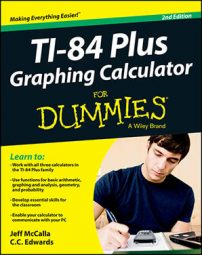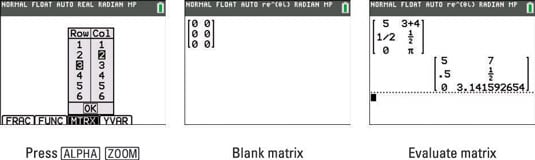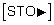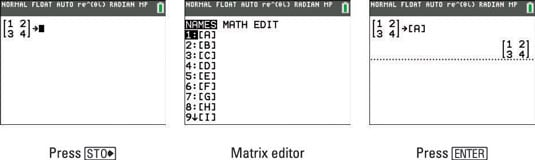##### Ti-84 Plus Graphing Calculator For DummiesYou can enter and store matrices on your TI-84 Plus calculator. A matrix is a rectangular array of numbers arranged in rows and columns. The dimensions, r x c, of a matrix are defined by the number of rows and columns in the matrix. The individual elements in a matrix are called elements.

## Entering matrices

Using the hidden MTRX shortcut menu is the preferred method of entering matrices (the easy way). Alternatively, you can use the Matrix editor found by pressing [2nd][x–1] (the hard way). Here are the instructions for entering matrices the easy way:

1. Press [ALPHA][ZOOM] to display the Quick Matrix Editor.

2. Use the arrow keys to highlight the dimensions you want and press [ENTER].

The default dimensions of a matrix are two rows by two columns. Here, a 3 x 2 matrix has been created.3. Use ; to highlight the word OK and then press [ENTER].

See the result in the second screen.

4. Enter an expression and press the right-arrow key to advance to the next element in the matrix.

Repeat this step until you have filled in every element in the matrix. When you press the right-arrow key after entering the last element in the first row, the calculator moves to the beginning of the second row and waits for you to make another entry.

To enter a fraction, delete the zero first and then press [ALPHA][Y=] to use the n/d fraction template.

5. Press [ENTER] to evaluate the matrix.

This is illustrated in the third screen.

You cannot copy and paste a matrix output from the calculator history. This is not a deal breaker! You may copy and paste the matrix expression you entered as many times as you would like.

## Storing a matrix

Storing a matrix is a handy feature to have around. This is especially helpful if you’re reusing the same matrix in future calculations. Follow these steps to store a matrix:

1. Enter a matrix on the Home screen.

See the preceding section for details — but don’t press [ENTER] yet!

2. Position your cursor to the right of your matrix and pressSee the first screen.

3. Press [2nd][x–1] and then press [ENTER] (to choose Matrix [A]).

Welcome to the Matrix editor. See the second screen.4. Finally, press [ENTER].

See the third screen.

You can delete a stored matrix by pressing [2nd][+] and then pressing [DEL] with your cursor on the matrix you want to delete. However, deleting a matrix is completely unnecessary. Saving a different matrix as [A] overwrites the current matrix [A].